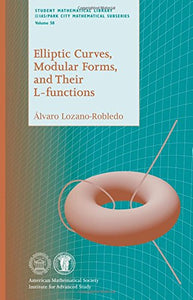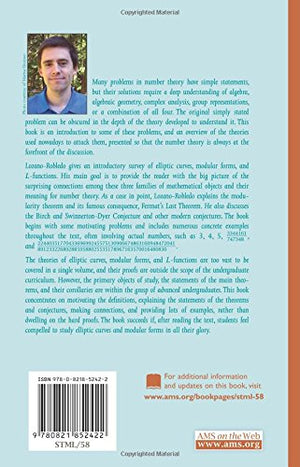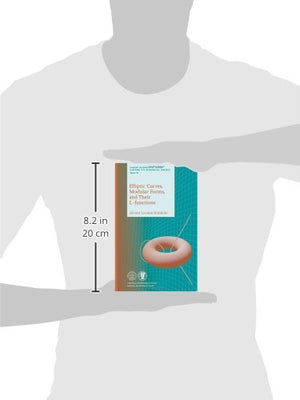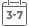# Elliptic Curves, Modular Forms, And Their L-Functions (Student Mathematical Library)

• Publish Date: 2011-02-08
• Binding: Paperback
• Author: lvaro Lozano-Robledo
Regular price $91.40 Sale price$62.79

Attention: For textbook, access codes and supplements are not guaranteed with used items.Many problems in number theory have simple statements, but their solutions require a deep understanding of algebra, algebraic geometry, complex analysis, group representations, or a combination of all four. The original simply stated problem can be obscured in the depth of the theory developed to understand it. This book is an introduction to some of these problems, and an overview of the theories used nowadays to attack them, presented so that the number theory is always at the forefront of the discussion. Lozano-Robledo gives an introductory survey of elliptic curves, modular forms, and $L$-functions. His main goal is to provide the reader with the big picture of the surprising connections among these three families of mathematical objects and their meaning for number theory. As a case in point, Lozano-Robledo explains the modularity theorem and its famous consequence, Fermat's Last Theorem. He also discusses the Birch and Swinnerton-Dyer Conjecture and other modern conjectures. The book begins with some motivating problems and includes numerous concrete examples throughout the text, often involving actual numbers, such as 3, 4, 5, $\frac{3344161}{747348}$, and $\frac{2244035177043369699245575130906674863160948472041} {8912332268928859588025535178967163570016480830}$. The theories of elliptic curves, modular forms, and $L$-functions are too vast to be covered in a single volume, and their proofs are outside the scope of the undergraduate curriculum. However, the primary objects of study, the statements of the main theorems, and their corollaries are within the grasp of advanced undergraduates. This book concentrates on motivating the definitions, explaining the statements of the theorems and conjectures, making connections, and providing lots of examples, rather than dwelling on the hard proofs. The book succeeds if, after reading the text, students feel compelled to study elliptic curves and modular forms in all their glory.